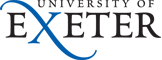Physics and Astronomy
Home Our Teaching Resources CDHW Feedback Answers.html

1. What does the circuit do and how does it work?
The circuit is a form of PID controller. The input signal is buffered and amplified by a non-inverting amplifier and the gain of this stage defines the proportional gain P of the controller. The amplified error signal passes in parallel through an integrator (top) a unity-gain amplifier (middle) and a differentiator (bottom) all of which have inverting behaviour. Their outputs are then summed and inverted by the final op-amp and passed to the output. The potentiometers labelled D and I control the proportions in which derivative and integral fractions contribute to the output signal which is proportional to the power W to be supplied to the heater.

2. What additional circuit is needed between the output and the heater?
A heater-driver that ensures that the power dissipated in the heater is proportional to the output voltage. This is usually either a square-rooter if the heater is to be driven by DC, or with AC heaters some type of pulse-width modulator.

3. Why is there a diode in series with the output?
The heater power can only be positive or zero so the diode keeps the output signal within these constraints.

4. Why might one want to make R2 much larger than R1?
A low value of R2 would 'load' the potentiometers and impair the linear relationship between control position and gain.

5. What is the purpose of R3?
The gain of a differentiator increases in proportion to frequency and without R3 it would only be limited by the, normally unnecessarily high, open-loop gain of the op-amp. R3 limits the gain at high frequencies reducing the noise of the system.

6. What might be the most serious effect of op-amp offset voltages currents and bias?
It is most likely to be troublesome by causing an offset between the set-point and oven temperatures. Under some circumstances the integrator may drift and eventually saturate which would prevent it from working properly.

7. How might the impact of op-amp offset and bias be reduced?
The first thing to try would be a resistor, equal in value to R2, between the positive input of the integrating op-amp and ground to eliminate the common-mode bias current. Selecting an op-amp with a good input-offset performance would be the next step.

8. How would you consider improving the circuit?
The improvements suggested in the previous answer would be a good start. The diode at the output could be replaced by an active rectifier for greater precision at low heater powers. Some method of reseting the integrator would help and maybe a circuit implement the 'Limit I?' function of the interactive simulation. Meters to indicate heater power and an error signal are helpful to the person who has to tune a controller for a particular oven.